# I2P_SUMMER

## June 19

#### Python:

This bit wasn’t too challenging for me. I watched a short video on if else statements and was set to finish.

To code this part I had a variable be the input of a prompt to see whether they wanted a circle or a triangle. After that I had an if else statement that checked if it was a circle. The else statement for that if has another if statement inside of it to see if its a triangle. If the initial input was either a circle or a triangle it was then asked for dimensions that would then output the final area of the shape.#### Javascript

Finished the rock paper scissors game activity, also started with the regular javascript course.

# June 20th

Managed to finish the daily task. After completing the codecademy section on functions it was relatively easy to complete. I did this by having a function return a random value when called upon. I then had two different variables be set to two random numbers. We then guess what the sum of those two might be and it prints whether we were right or wrong.# June 21st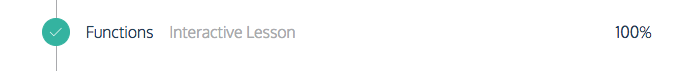The codecademy units I completed today

#### Python:

To make the add delete and update functions of the calendar app we were supposed to make I set up one delete function, three edit functions, three add functions, however I could have definitely done this in a single add  function and a single edit function. I also made three lists, each storing month day and name of the event.I set up the lists dates, months, and names to store their respective values.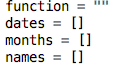So to add to the calendar I set up three functions that each add to an individual list, however it would have been a lot simpler to have one add function to add to all three. When the program runs it asks if the user would like to add edit or update. If they would like to add it asks what the name date and month of the event will be.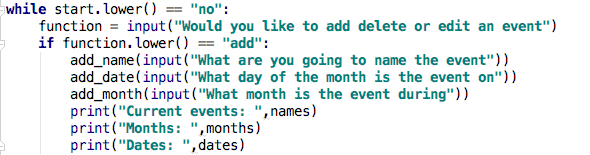To edit a function if the user inputs the word edit, it prints off all of the current events and asks for the name of the event to edit.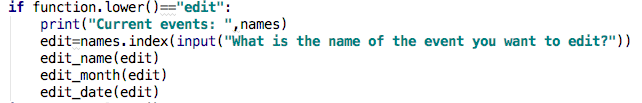This was the actual edit functions, I could have easily just stitched these together into one, longer edit function.

def
edit_name(name):
names[name]=input(“What is the new name?”)

def edit_date(date):
dates[date]=input(“What is the new date?”)

def edit_month(month):
months[month]=input(“What is the new month?”)

To delete an event I would use this segment of code.

if function.lower()==“delete”:
print(“Current events: “,names)
print(“Months: “,months)
print(“Dates: “,dates)
delete(names.index(input(“What is the name of the event you want to delete?”)))

This was the singular delete function

```def delete(index):
del(dates[index])
del(names[index])
del(months[index])```

To keep the program running forever I had a variable called start that was set to ‘no’.

while start.lower() == “no”:
“all my code”

At the bottom of the loop it asks whether the user is finished and if they type yes I use break to end the program. If it is ‘no’ or anything else it resets the cycle and the user can continue to edit the calendar.

#### Javascript

In the building a calendar segment of the javascript course we learned to use a lot about lists and objects.

var object = {
trait1 = 2,
trait2 = 3
};

list = [object, ect, ect…];

We also learned how to call certain traits from an object through the list. For example this would print through as 2.

console.log(list[object.trait1);

We learned how you can have lists full of objects that each have their own individual traits. We also learned to use a function to create new dynamically made objects and add them to lists.

var newperson = {
firstName:firstname,
lastName:lastname,
phoneNumber:phone,
email:mail};
contacts[contacts.length]=newperson;
}

Overall we learned about lists, reviewed using for loops, and learned more about the things you can do in functions.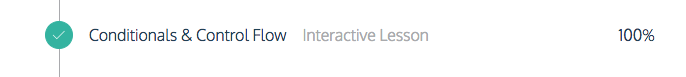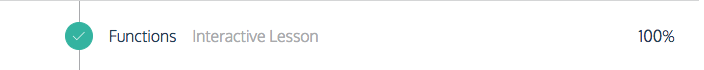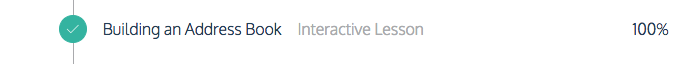The codecademy units I completed today

# June 22,

#### Python:

For this I used these sources:

https://stackoverflow.com/questions/3387655/safest-way-to-convert-float-to-integer-in-python

http://effbot.org/pyfaq/how-do-i-generate-random-numbers-in-python.htm

My code:

I generated a random number using random.random() and had it be returned in function rand(). I then set a variable to the user guess using input(guess). I used a while loop that would either tell you you your guess was to high or low while adding to the guesses variable. Then when you eventually get the right answer it prints: “you have won in”, guesses,”attempts!” This was pretty straightforward however I learned that there is a random python module and about the floor() function.

import random
import pygame
import math

def rand():
return math.floor(random.random()*100)

guesses = 0;
computer = rand()
guess=int(input(“Guess a number between 1 and 100”))

while guess != computer:
if guess > computer:
guess=int(input(“Guess a number between 1 and 100”))
guesses=guesses+1
if guess < computer:
guess=int(input(“Guess a number between 1 and 100”))
guesses=guesses+1

if guess == computer:
print(“You won in”,guesses,“attempts!”)

# Javascript

I used these websites: https://stackoverflow.com/questions/596467/how-do-i-convert-a-float-number-to-a-whole-number-in-javascript

My code

```var guess = prompt("Guess a number between 1 and 100")
var computer = Math.floor(Math.random()*100);```
```while(guess != computer) {
if(guess > computer) {
var guess = prompt("Guess a number between 1 and 100")
}
if(guess < computer) {
var guess = prompt("Guess a number between 1 and 100")
}
}```

console.log(“You won!”);

How it works:

This is actually pretty simple. First I define two variables, one being the user guess using prompt(). The other variable is the computer generated number, this is the new things I learned. I learned how to use Math.floor() to simplify simplify Math.random*100. This gives me a clean, whole, number that is between 1 and 100. After this It was a simple while loop that runs as long as guess != computer. If guess is larger than the computer it says your guess and if it is too low it says your guess is too low. Once you correctly guess the number and the loop ends we log “You won!” to the console. Overall very simple.

Codecademy completed: I did this challenge the same day as the 21st.

# June 23rd

Python:

To make the encryptor I wanted to avoid copy pasting lines of .replace(a,z) so I tried to make a short and concise function that would translate from the regular alphabet to a cryptokey.

#### This was my original attempt at making a simple encryptor

```def encrypt(message):
alphabet = [" ","a","b","c","d","e","f","g","h","i","j","k","l","m","n","o","p","q","r","s","t","u","v","w","x","y","z"]
key = [" ","4","x","z","@","%","b","j","q","(","ƒ","¥","µ","˚","nå","ø","π","å","œ","¢","∞","∫","µ","≈","`","¬","…"]
new_message = ""
for x in range(0,len(message)):
new_message = message.replace(message[x],key[alphabet.index(message[x])])
return new_message

print(encrypt(input("What would you like to encrypt").lower()))

```

This code however, just didn’t work and wouldn’t replace every character in the original string.

After posting this query on stackoverflow I was presented with a range of other ways to accomplish my goal.

In the end I learned how to use the translate() function to encrypt a user input.

```def encrypt(message):
new = message.lower()
alphabet = 'abcdefghijklmnopqrstuvwxyz'
key = '4xz@%bjq(ƒ¥µ˚nåøπåœ¢∞∫µ≈`¬'
table = str.maketrans(alphabet, key)

return new.translate(table)

print(encrypt('Hi my name is thomas')) #this prints: q( ˚` n4˚% (œ ¢qå˚4œ```

This chunk of code basically takes the two strings alphabet and key and makes a translate table called table. We then use the translate() function to translate our message.

#### Completed Code

```def encrypt(message):
new = message.lower()
alphabet = 'abcdefghijklmnopqrstuvwxyz'
key = '4xz@%bjq(ƒ¥µ˚nåøπåœ¢∞∫µ≈`¬'
table = str.maketrans(alphabet, key)

return new.translate(table)

def decrypt(message):
new = message.lower()
alphabet = 'abcdefghijklmnopqrstuvwxyz'
key = '4xz@%bjq(ƒ¥µ˚nåøπåœ¢∞∫µ≈`¬'
table = str.maketrans(key, alphabet)

return new.translate(table)

if input("Do you want to decrypt").lower() == "yes":
print(decrypt(input("What would you like to decrypt")))

if input("Do you want to encrypt").lower()=="yes":
print(encrypt(input("What would you like to encrypt")))```

Console output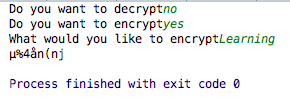#### Alternative version:

I also found another solution to my original problem using dictionaries.

```encryption_dict = dict(zip(alphabet, key))
decryption_dict = dict(zip(key,alphabet))

if input("Would you like to encrypt or decrypt").lower() == "encrypt":
my_str = input("What would you like to encrypt").lower()
new_str = ''.join(encryption_dict.get(s, s) for s in my_str)
print(new_str)
else:
my_str = input("What would you like to decrypt").lower()
new_str = ''.join(decryption_dict.get(s, s) for s in my_str)
print(new_str)

```

This was more me ripping from my stackoverflow question earlier, and I am not totally sure about how it works. Hence why I used translate() as my final version.

This was a pain to do and am still working on it right now.

# Weekend Challenge

#### What I chose:

I chose to complete the applying to HKIS challenge. To explain it basically, the application form calls a function that returns a list containing all of your childs info, this is then added to an applications list and the user is given the option to add another child.

`applications = []`

new = []
new.append(input(“What is the first name of your child?”))
new.append(input(“What is the last name of your child?”))
new.append(input(“Male or Female?”))
new.append(input(“Date of birth (mm/dd/yyyy)”))
new.append(input(“Have your child answer, why do you want to come to this school”))
#More application questions
return new

#### How it works

This function is actually somewhat simple. It creates a temporary list inside the function that contains all of the input data such as name and birthdate. This is then returned and can be added to the applications list containing all of the applications.

#### The rest of the code:

start = 1
while start == 1:
if input(“Would you like to add another child?”).lower() == “yes”:
if input(“Are you finished”).lower()==“yes”:
print(applications)
print(“We will send an acceptance letter regarding you child(s) within the next two months”)
break

Console output: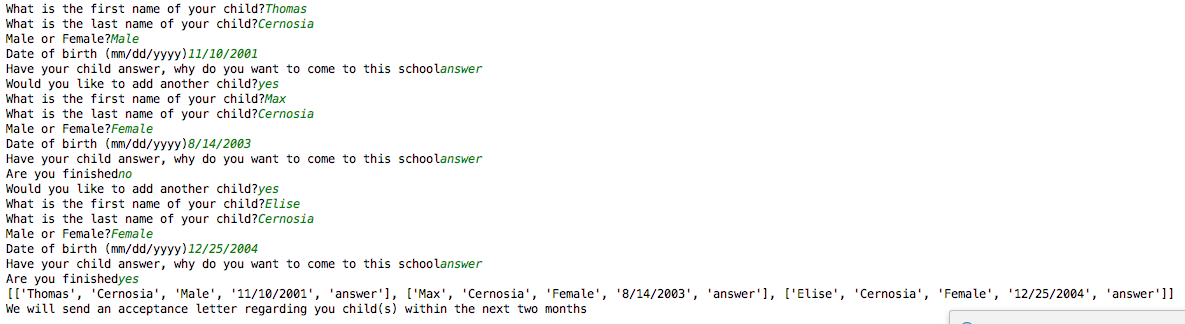# Mini Project (week two)

I chose to make a hangman game. This is the finished code

```import random
import pygame

current = list(random.choice(words))
placeholder = "____________________"
new_placeholder = list(placeholder[:len(current)])
guesses = []
print("You have 5 lives to guess the word")
print(new_placeholder)
lives = 10

while new_placeholder != current:
guess = input("What letter do you want to guess?").lower()
if guess in current:
hold = current.index(guess)
new_placeholder[hold] = guess
print("Correct! \n",new_placeholder)
else:
lives -= 1
print("Incorrect!\nYou have ",lives," lives left")

print("You won with ",lives," lives left!")```

Its actually very simple to understand. I have a small database of words that are turned into a list. I have a variable called placeholder that is all underlines. This is shortened to the length of the random word that is chosen. If the user guess matches a letter in the hidden word list it modifies the placeholder list. The while loop ends when new_placeholder == the hidden word.

# Final Project Check in 1

Progress So Far:

I have just completed both the fight and enemy functions. Along with basic completion for the rest of the game. I am almost complete with the game prototype.

Current Code:

```import random
import pygame
import math
from time import sleep

def enemy():
demon = {"name":"Demon","health":200,"attack":50,"armor":20,"accuracy":40}
monkey = {"name":"Monkey","health":50,"attack":10,"armor":0,"accuracy":70}
goblin = {"name":"Goblin","health":100,"attack":30,"armor":10,"accuracy":70}
mega_demon = {"name":"Mega Demon","health":500,"attack":150,"armor":30,"accuracy":50}
enemies = [demon,monkey,goblin,mega_demon]
return random.choice(enemies)

#player creation

player = {'name': input("What is your name"),"health":200,"armor":0,"accuracy":90,"attack":25}
print("Welcome",player["name"])
input("press any key to proceed")
print("Have a total of 20 stat points to spend on armor, health, accuracy, and attack")
print("1 point into health adds 10 hp to your character\n1 point into armor reduces damage taken by 1\n1 point  into accuracy increases hit chance by 1% (default 90%)\n1 point into attack increases damage dealt by 5")
health = 0
armor = 0
accuracy = 0
attack = 0

while health + armor + accuracy + attack < 20:
health = int(input("how many stat points would you like to add to health"))
armor = int(input("how many stat points would you like to add to armor"))
accuracy = int(input("how many stat points would you like to add to accuracy"))
attack = int(input("how many stat points would you like to add to attack"))

player["health"] += health * 10
player["armor"] += armor * 5
player["accuracy"] += accuracy
player["attack"] += attack * 5

print(player)
#introduction to dungeon
print("\nWelcome " + player["name"] + ", you stand outside a dungeon, you have to reach the sacred alter and retrieve an artifact\nThe dungeon is filled with traps and strange enemies that you must fight through\ngood luck!")```

```def fight(player,current_enemy):
print("You found a ",current_enemy["name"])
sleep(3)
while player["health"] >= 0 and current_enemy["health"] >= 0:
if math.floor(random.random()*100) >= current_enemy["accuracy"]:
print("The enemy missed!")
sleep(1)
else:
print("You take ",current_enemy["attack"]," damage")
player["health"]-=current_enemy["attack"]

```

The fight function is nearly complete. Just need to copy and paste the enemy and change it to fit the character.

#### Next steps:

Complete the fight() function as well as the room() function and the run loop to tie the game together. Also just general code revisions and polishing.

# Final Project Checkpoint 2

Progress so far:

Completed the basic frame of the game. Added the run loop and completed the room() function for moving around.

#### The run loop:

```boss_dead = 0

if current_room == "boss":
fight(player,boss = {"name":"Mega demon","health":500,"attack":150,"armor":30,"accuracy":50})
break
else:
current_enemy = enemy()
fight(player,current_enemy)
current_room = rooms(current_room)

```

The room() function:

```def rooms(current):
entrance = ["left","right"]
left_one = ["forward","right"]
right_one = ["left","forward"]
left_path = ["forward"]
right_path = ["forward"]
all_rooms = [entrance,left_one,right_one,left_path,right_path]

if current == "entrance":
print("You can move: ", entrance)
hold = input("What do you want to do?").lower()
while (hold != "left") and (hold != "right"):
hold = input("What do you want to do?").lower()
if hold == "left":
print("You walk into the door on the left")
return "left_one"
if hold == "right":
print("You walk into the door on the right")
return "right_one"
if current == "left_one":
print("You can move: ", left_one)
hold = input("What do you want to do?").lower()
while hold != "forward" and hold != "right":
hold = input("What do you want to do?").lower()
if hold == "left":
print("You walk down the winding left path")
return "left_path"
if hold == "right":
print("You walk into the room on the right")
return "right_one"
if current == "right_one":
print("You can move: ", right_one)
hold = input("What do you want to do?").lower()
while hold != "left" and hold != "forward":
hold = input("What do you want to do?").lower()
if hold == "left":
print("You walk into the room on the left")
return "left_one"
if hold == "right":
print("You walk down the winding path forward")
return "right_path"
if current == "left_path":
print("You can move: ", left_path)
hold = input("What do you want to do?").lower()
while hold != "forward":
hold = input("What do you want to do?").lower()
print("You walk out of the narrow passage into an open, dimly lit room")
return "boss"
if current == "right_path":
print("You can move: ", right_path)
hold = input("What do you want to do?").lower()
while hold != "forward":
hold = input("What do you want to do?").lower()
print("You walk out of the narrow passage into an open, dimly lit room")
return "boss"
current_room = "entrance"

```

This function is what will control character movement in the game.

#### Stuff to work on next:

Next I just need to maybe revise the run loop to make something more interactive. I also just need to wrap up and polish code/revise certain parts like in the room() loop to make it more efficient.

```
```